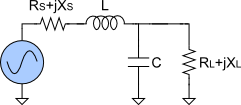# Impedance Matching Calculator

Created by Wojciech Sas, PhD candidate
Reviewed by Steven Wooding
Last updated: Aug 10, 2021

This is the impedance matching calculator - a great tool that helps you solve problems with matching electronic elements within a circuit. In the article, you can find a short explanation of the concept of impedance matching and learn three basic impedance matching topologies (L-match, Pi-match, and T-match).

## The meaning of impedance matching

The impedance matching is a common concept in electronics that helps design a circuit that maximizes the power transfer and/or minimizes signal reflection from the load.

In general, we have a source of the signal (radio transmitter, generator), and we want to transmit that signal to a load (antenna, speaker, or just a transmission line). Each of them has a characteristic impedance - a complex quantity that describes the opposition to the current flow, both static (resistance, denoted as R) and dynamic (reactance, denoted as X).

We can ensure the maximal power transfer if the load impedance is equal to the source impedance's complex conjugate. For only resistive components, it means that the source's resistance should be equivalent to the load's resistance. In practice, we usually deal with circuits that also have specific reactance components, so that's why we need a tool that tells us how to the arrange electric elements.

So, how do you match an impedance or solve a circuit? In short - you need to find a capacitance and an inductance (sometimes a few of them) so that the condition for the maximal power transfer is valid. The exact values depend on the topology of the system and the current type.

Although there are many possible ways to match impedance in a system, we provide three basic circuit topologies in our impedance matching calculator, namely L-match, Pi-match, and T-match.

## Three basic impedance matching topologies

To obtain a result, you should enter the following quantities:

• The values of resistances (RS, RL) and reactances (XS, XL) of the source and the load;

• The frequency (F) of the signal; and

• The possibility/suppression to pass direct current. The configuration that passes DC is lowpass, and the corresponding one is a highpass match.

Sometimes you'll also need to input the quality factor (Q).

### L-match circuit

The first of the systems is the L-match circuit. The name comes from the fact that two elements, an inductor, and a capacitor, align in a letter L. If you've ever heard about voltage dividers, the configuration may look familiar.

As a result, you get the values of the inductance (L), the capacitance (C), and the output Q factor.

### Pi-match circuit

The next circuit is Pi-match, as three components in the middle resemble the Greek capital letter Π.

• For the lowpass system, you receive the values of the inductance (L), source capacitance (CS), and load capacitance (CL).

• For the highpass system, you receive the values of the capacitance (C), source inductance (LS), and load inductance (LL).

### T-match circuit

The last of the configurations is T-match. At this point, you shouldn't be surprised that matching elements form a letter T, should you?

• For the lowpass system, you receive the values of the capacitance (C), source inductance (LS), and load inductance (LL).

• For the highpass system, you receive the values of the inductance (L), source capacitance (CS), and load capacitance (CL).

## How to use this impedance matching calculator?

Our tool is pretty straightforward to use. The only thing you need to type is input parameters, and you'll get the results immediately. Take a look at an example:

1. Choose the type of your circuit. Let it be Pi-Match.

2. Set the frequency to 110 MHz.

3. Enter source resistance and capacitance, which are 50 Ω and 0 Ω, respectively.

4. Repeat the same for load inputs - 150 Ω for the resistance and 0 Ω for the reactance.

5. Type input Q factor as 2.5.

6. Disable passing DC (Block DC Current option).

And that's all! Your outcome values should be:

• 18.95 pF for the capacitance.

• 60.78 nH for the source inductance.

• 86.81 nH for the load inductance.

Wojciech Sas, PhD candidateCircuit type
L-Match
Frequency (F)
MHz
Source resistance (RS)
Ω
Source reactance (XS)
Ω
Ω
Ω
Circuit DC current
Pass DC Current
Results
Inductance (L)
79.58
nH
Capacitance (C)
15.915
pF
Q factor
1
Charts
Show plot?
Real & Imag
Note that the values on frequency x-axis are shown using the logarithmic scale.
People also viewed…

### Audio file size

This audio file size calculator will help you estimate how much space an uncompressed audio file will take up. You will also learn about audio bit depth, sample rate, and more stuff about digital audio. Keep tuned in to learn more.

### Humans vs vampires

Vampire apocalypse calculator shows what would happen if vampires were among us using the predator - prey model.

### Plastic Footprint

Find out how much plastic you use throughout a year with this plastic footprint calculator. Rethink your habits, reduce your plastic waste, and make your life a little greener.

### Semitone

Use the semitone calculator to determine the distance between two frequencies.